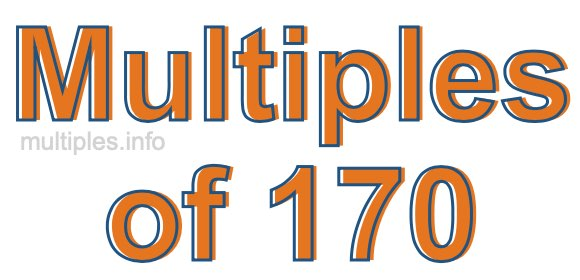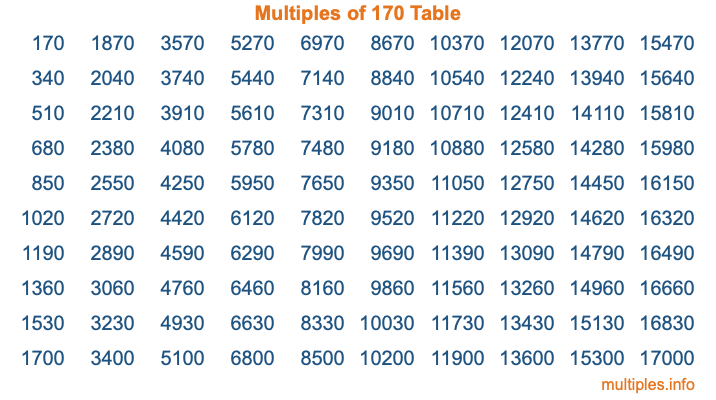Multiples of 170Welcome to the Multiples of 170 page. Here we will first teach you everything you will ever need to know about the multiples of 170, and then give you a study guide summary of everything we taught you to make sure you remember it all. Use this page to look up facts and learn information about the multiples of 170. This page will make you a multiples of one hundred seventy expert!

Definition of Multiples of 170
Multiples of 170 are all the numbers that when divided by 170 equal an integer. Each of the multiples of 170 are called a multiple. A multiple of 170 is created by multiplying 170 by an integer.

Therefore, to create a list of multiples of 170, you start with 1 multiplied by 170, then 2 multiplied by 170, then 3 multiplied by 170, and so on for as long as you want. Thus, the list of the first five multiples of 170 is 170, 340, 510, 680, and 850. To see a larger list of multiples of 170, see the printable image of Multiples of 170 further down on this page. We also have a category where you can choose any nth multiple of 170.

Multiples of 170 Checker
The Multiples of 170 Checker below checks to see if any number of your choice is a multiple of 170. In other words, it checks to see if there is any number (integer) that when multiplied by 170 will equal your number. To do that, we divide your number by 170. If the the quotient is an integer, then your number is a multiple of 170.

Is  a multiple of 170?

Least Common Multiple of 170 and ...
A Least Common Multiple (LCM) is the lowest multiple that two or more numbers have in common. This is also called the smallest common multiple or lowest common multiple and is useful to know when you are adding our subtracting fractions. Enter one or more numbers below (170 is already entered) to find the LCM.

Check out our LCM Calculator if you need more details about the Least Common Multiple or if you need the LCM for different numbers for adding and subtraction fractions.

nth Multiple of 170
As we stated above, 170 is the first multiple of 170, 340 is the second multiple of 170, 510 is the third multiple of 170, and so on. Enter a number below to find the nth multiple of 170.

th multiple of 170

Multiples of 170 vs Factors of 170
170 is a multiple of 170 and a factor of 170, but that is where the similarities end. All postive multiples of 170 are 170 or greater than 170. All positive factors of 170 are 170 or less than 170.

Below is the beginning list of multiples of 170 and the factors of 170 so you can compare:

Multiples of 170: 170, 340, 510, 680, 850, etc.

Factors of 170: 1, 2, 5, 10, 17, 34, 85, 170

As you can see, the multiples of 170 are all the numbers that you can divide by 170 to get a whole number. The factors of 170, on the other hand, are all the whole numbers that you can multiply by another whole number to get 170.

It's also interesting to note that if a number (x) is a factor of 170, then 170 will also be a multiple of that number (x).

Multiples of 170 vs Divisors of 170
The divisors of 170 are all the integers that 170 can be divided by evenly. Below is a list of the divisors of 170.

Divisors of 170: 1, 2, 5, 10, 17, 34, 85, 170

The interesting thing to note here is that if you take any multiple of 170 and divide it by a divisor of 170, you will see that the quotient is an integer.

Multiples of 170 Table
Below is an image of the first 100 multiples of 170 in a table. The table is in chronological order, column by column. The first column has the first ten multiples of 170, the second column has the next ten multiples of 170, and so on.The Multiples of 170 Table is also referred to as the 170 Times Table or Times Table of 170. You are welcome to print out our table for your studies.

Negative Multiples of 170
Although not often discussed or needed in math, it is worth mentioning that you can make a list of negative multiples of 170 by multiplying 170 by -1, then by -2, then by -3, and so on, to get the following list of negative multiples of 170:

-170, -340, -510, -680, -850, etc.

Multiples of 170 Summary
Below is a summary of important Multiples of 170 facts that we have discussed on this page. To retain the knowledge on this page, we recommend that you read through the summary and explain to yourself or a study partner why they hold true.

There are an infinite number of multiples of 170.

A multiple of 170 divided by 170 will equal a whole number.

170 divided by a factor of 170 equals a divisor of 170.

The nth multiple of 170 is n times 170.

The largest factor of 170 is equal to the first positive multiple of 170.

170 is a multiple of every factor of 170.

170 is a multiple of 170.

A multiple of 170 divided by a divisor of 170 equals an integer.

170 divided by a divisor of 170 equals a factor of 170.

Any integer times 170 will equal a multiple of 170.

Multiples of a Number
Here you can get the multiples of another number, all with the same attention to detail as we did for multiples of 170 on this page.

Multiples of
Multiples of 171
Did you find our page about multiples of one hundred seventy educational? Do you want more knowledge? Check out the multiples of the next number on our list!